Minkowski Metric:

Stills:

800x800 resolution, time = 0.4 M
 The pressure profile of the rigid rotor using hyperbolic divergence cleaning. The B2 profile of the rigid rotor using hyperbolic divergence cleaning.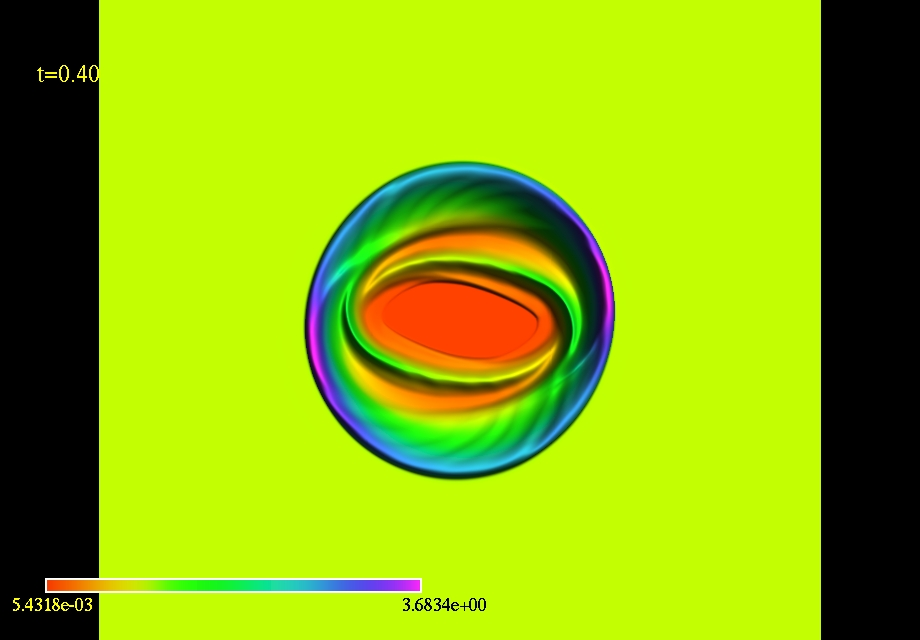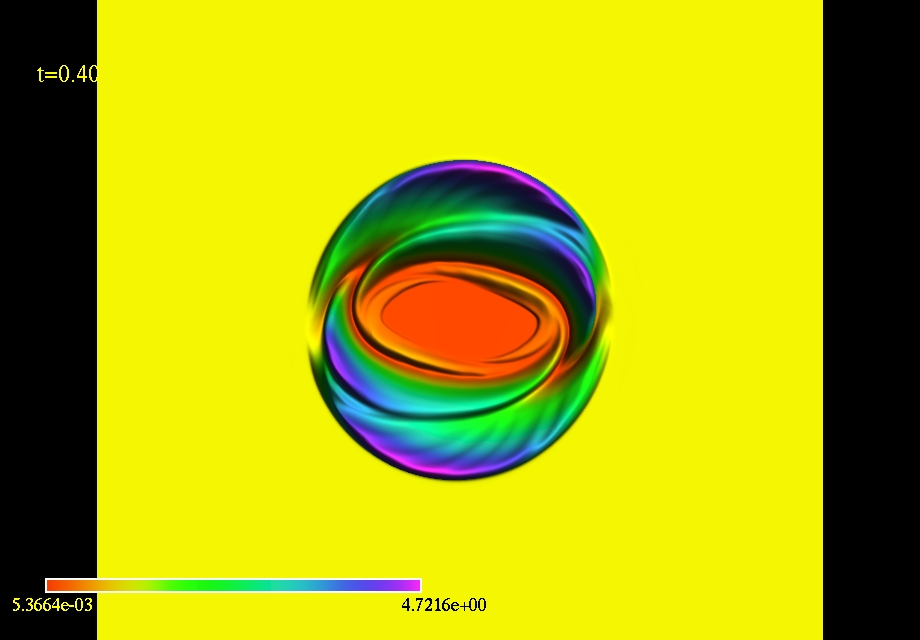2400x2400 resolution, time = 4.0 M
 The Bx profile of the cylindrical explosion using hyperbolic divergence cleaning. The By profile of the cylindrical explosion using hyperbolic divergence cleaning.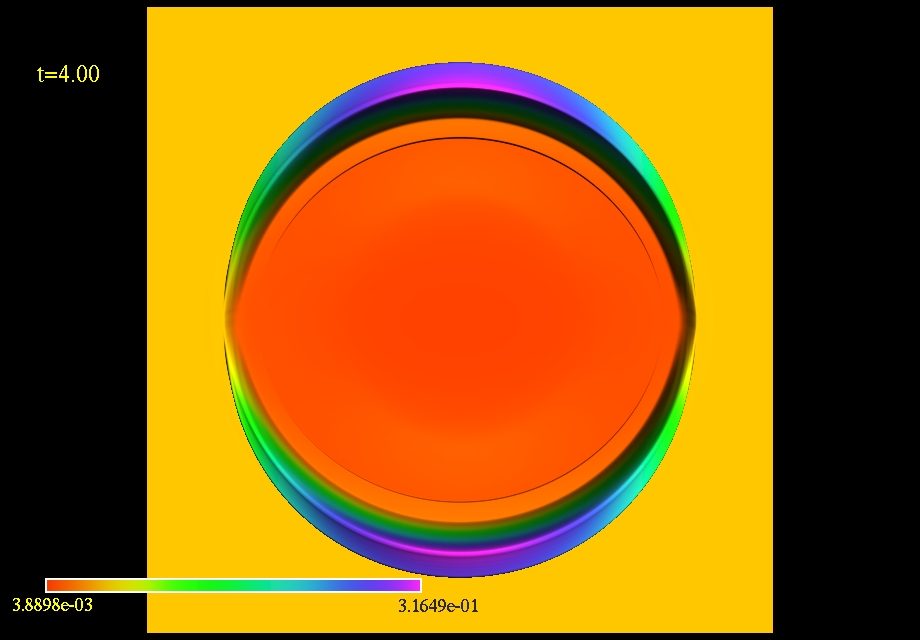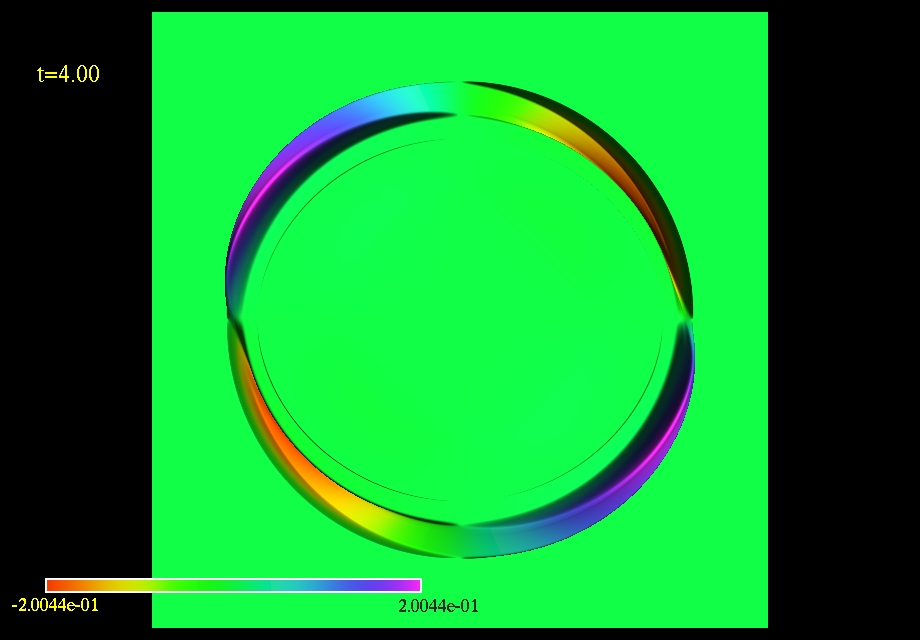256x256 resolution, time = 15.0 M
 The density profile of the magnetized Kelvin--Helmholtz instability using hyperbolic divergence cleaning. Bx = 0.5 The density profile of the magnetized Kelvin--Helmholtz instability using hyperbolic divergence cleaning. Bx = 5.0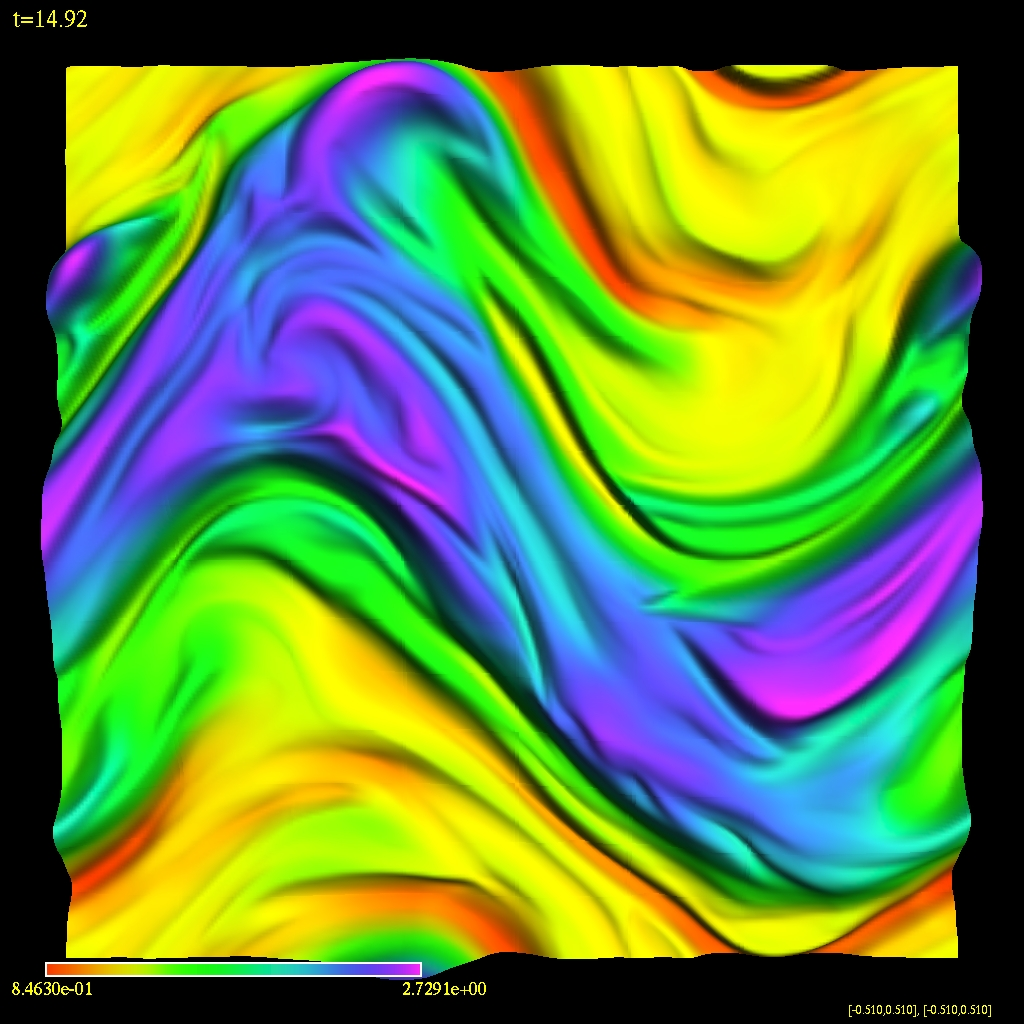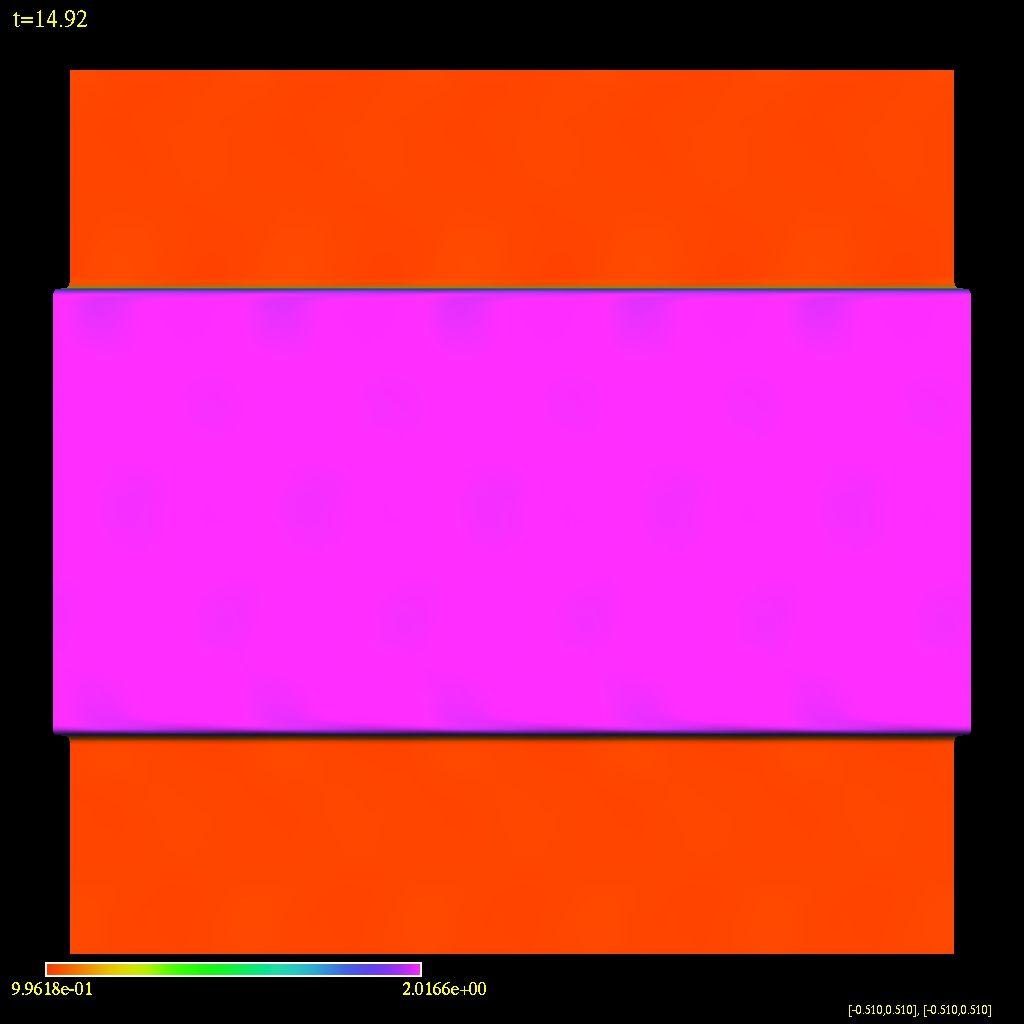The density profile of the magnetized Kelvin--Helmholtz instability using hyperbolic divergence cleaning. By = 0.5 The density profile of the magnetized Kelvin--Helmholtz instability using hyperbolic divergence cleaning. By = 5.0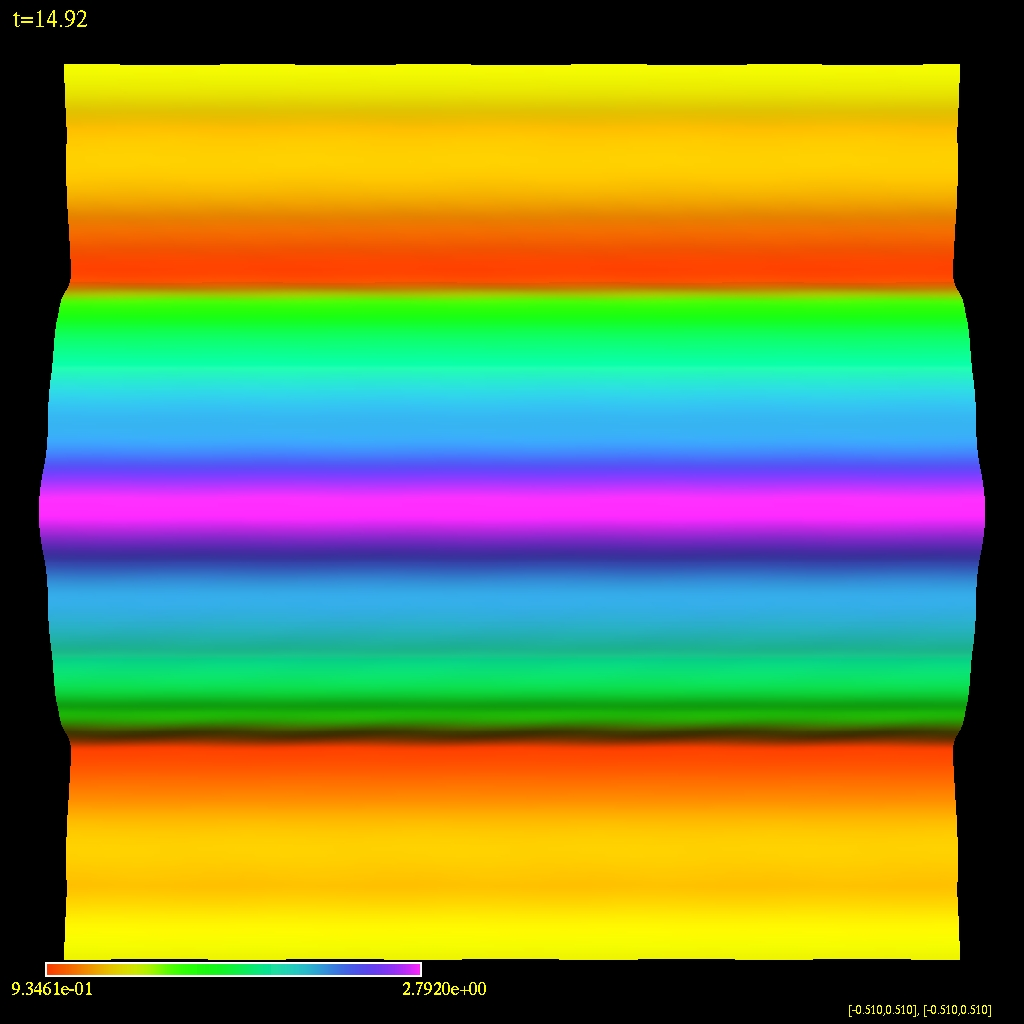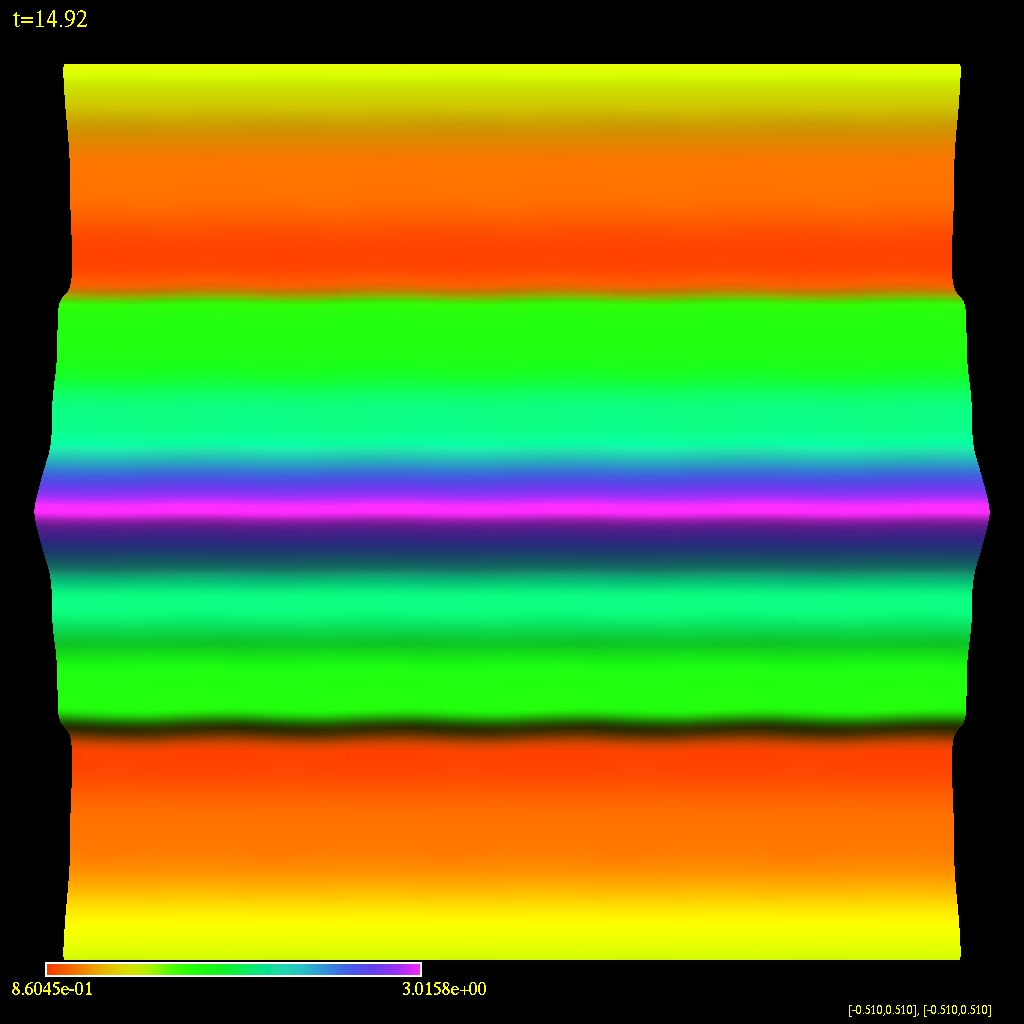Non-magnetized KHI
 The density profile of the hydrodynamic Kelvin--Helmholtz instability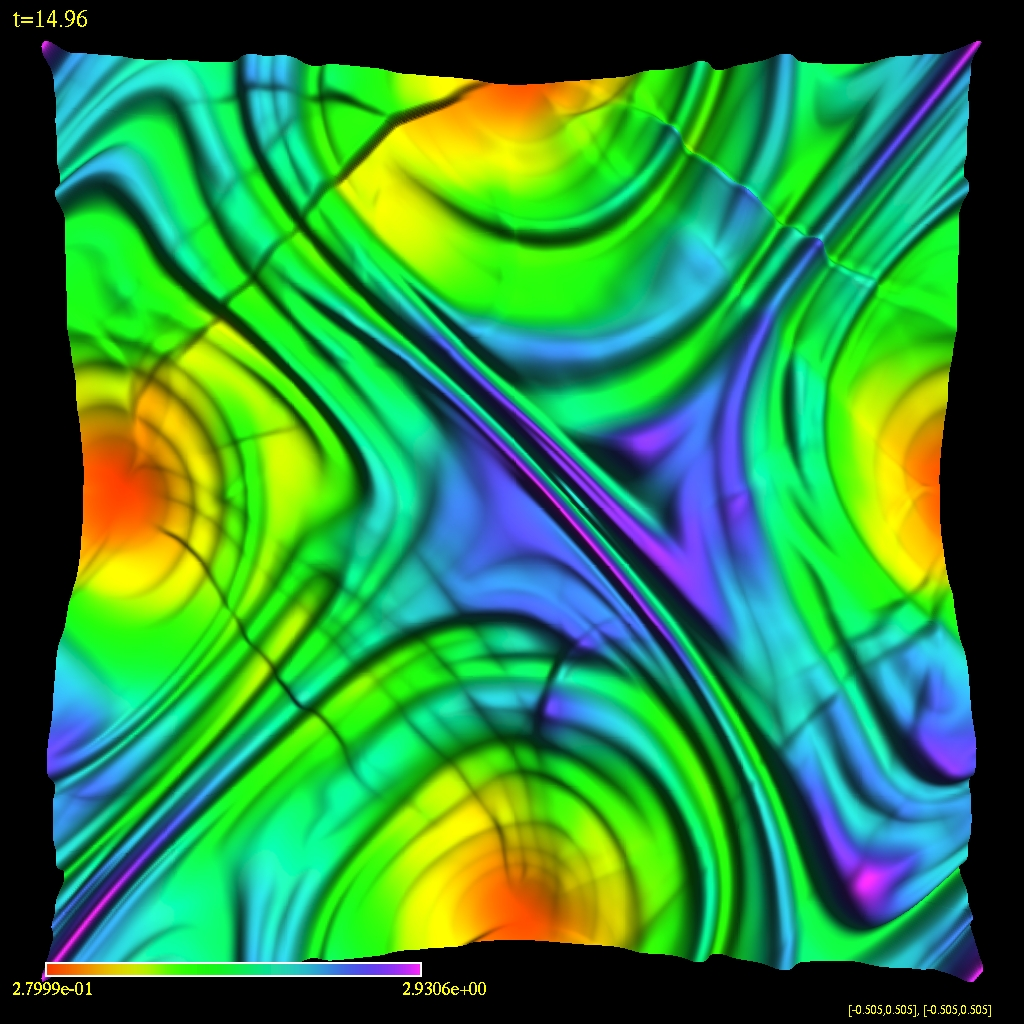Cartesian Coordinates:

B Field   No B Field
Shock Test Blast Wave KHI pert V KHI pert B Relativistic Rotor 256 Orszag-Tang Rotor 128 Rotor 256 KHI
Pressure Pressure Density, Bx=0.5 Density, Bx=0.5 Pressure Pressure Pressure Pressure Density
Density Bx Density, Bx=5 Density Bx=5 |v| Density |v| |v|
Vorticity By Density, By=0.5   |B| Speed |B| |B|
Density Density, By=5.0   Density   Density Density

Schwarzschild Metric:

Schwarzschild Coordinates:

1D Steady State Solution

Static Regular GridRegular GridLograrithmic Grid Regular Grid Convergence
Density Density Density Density
Pressure Presure Pressure
Radial Velocity Radial velocity Radial Velocity

Ingoing Eddington Finkelstein Coordinates:

1D Steady State Solution:

Regular GridLograrithmic Grid
Density Density
Presure Pressure
Radial velocity Radial Velocity

2D Hydrodynamic Steady State Solution:

Single Processor Multiprocessor
Static, Regular Grid Dynamic, Regular Grid
Radial Velocity Radial Velocity
Radial Velocity Top View Radial Velocity Bounding Box
Density Density
Pressure Pressure

2D Hydrodynamic Axisymmetric Evolution:

Bondi-Hoyle Accretion:

M=1
Logarithmic Density
Radial Velocity
Angular Velocity

Equatorial Hydrodynamic Non-Axisymmetric Evolution:

Bondi-Hoyle Accretion:

Half Plane Full Plane
M=1 M=1
Logarithmic Density Logarithmic Density
Radial Velocity

Ultra Relativistic Bondi-Hoyle Accretion:

M=1, &Gamma=4/3, c=&radic&Gamma

v=0.6
Logarithmic Pressure
Logarithmic Energy

Magnetized Relativistic Bondi-Hoyle Accretion:

M=1, &Gamma=4/3, c=0.1

v=0.6
Logarithmic Density
Logarithmic Radial Magnetic Field

Kerr Metric:

Boyer-Lindquist Coordinates:

2D Hydrodynamic Axisymmetric Evolution Kerr:

Bondi-Hoyle Accretion:

M=1,a=1
Logarithmic Density
Radial Velocity
Angular Velocity

Maintained by ajpenner@physics.ubc.ca. Supported by Matt Choptuik